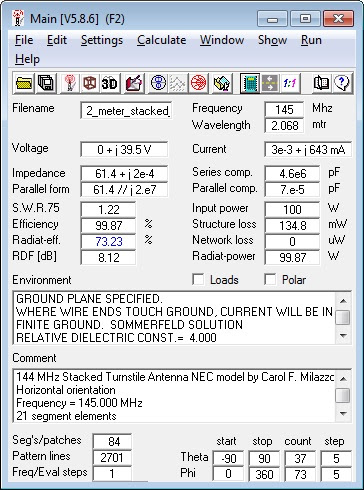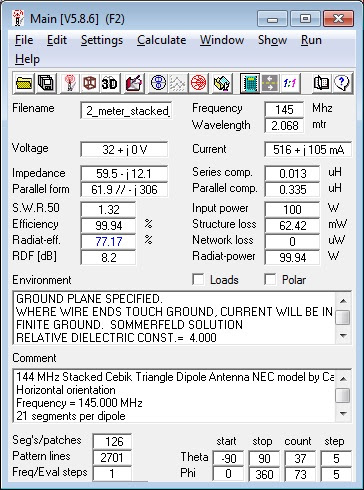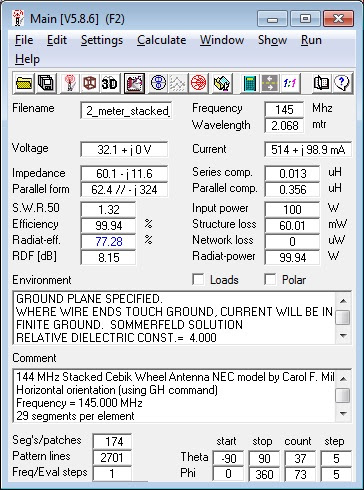# 144 MHz Omnidirectional Horizontal Antennas - Part 6

## NEC Model Comparisons of Stacked Turnstile and Cebik Triangle and Wheel Omnidirectional Horizontally Polarized Antennas for 144 MHz

by Dr. Carol F. Milazzo, KP4MD (posted 05 January 2013)
E-mail: [email protected]

### INTRODUCTION

Among horizontally polarized antennas used for weak signal VHF terrestrial communications, Cebik4 analyzed the Big Wheel, an omnidirectional array of three full wave loops, as a 1.5λ circular radiator fed at high impedance points through three radial spoke parallel transmission lines.  He proposed as an alternative design an array of three discrete dipoles fed at their low impedance points, either in an equilateral triangle or curved into a circle.

This study uses NEC modeling to compare the expected performance of each of these antenna designs against a turnstile antenna.  The antennas were modeled with 4nec2 at 0.5λ and 1λ above a simulated ground.

1. 144 MHz stacked Turnstile Antenna horizontal polarization radiation pattern calculated by NEC Model.

2. 144 MHz stacked Cebik Triangle Antenna horizontal polarization radiation pattern calculated by NEC Model.

3. 144 MHz stacked Cebik Wheel Antenna horizontal polarization radiation pattern calculated by NEC Model.

4. 144 MHz stacked Turnstile Antenna vertical polarization radiation pattern calculated by NEC Model.

5. 144 MHz stacked Cebik Triangle Antenna vertical polarization radiation pattern calculated by NEC Model.

6. 144 MHz stacked Cebik Wheel Antenna vertical polarization radiation pattern calculated by NEC Model.

7. 144 MHz stacked Turnstile Antenna azimuth pattern calculated by NEC Model.

8. 144 MHz stacked Cebik Triangle Antenna azimuth pattern calculated by NEC Model.

9. 144 MHz stacked Cebik Wheel Antenna azimuth pattern calculated by NEC Model.

10. 144 MHz stacked Turnstile Antenna elevation pattern calculated by NEC Model.

11. 144 MHz stacked Cebik Triangle Antenna elevation pattern calculated by NEC Model.

12. 144 MHz stacked Cebik Wheel Antenna elevation pattern calculated by NEC Model.14. 144 MHz stacked Turnstile Antenna 4nec2 Calculations.14. 144 MHz stacked Cebik Triangle Antenna 4nec2 Calculations.15. 144 MHz stacked Cebik Wheel Antenna 4nec2 Calculations.

CM 144 MHz Stacked Turnstile Antenna NEC model by Carol F. Milazzo, KP4MD
CM Horizontal orientation
CM Frequency = 145.000 MHz
CM 21 segments per dipole
CM Simulated good ground
CM http://www.qsl.net/kp4md/omnihoriz.htm
CE
SY frq=145    'Input frequency MHz
SY len=39.815    'Input dipole length inches
SY dia=0.25    'Input dipole dia. inches
SY rad=0.5*dia    'Calculate dipole radius
SY n=21    'Input segments per dipole (must be odd)
SY nc=0.5*(n+1)    'Calculate feed point segment
SY h=40.87    'Input height inches to 0.5 lambda
SY s=0.25    'Input separation between each dipole in pair inches
GW    1    n    0    -len/2    h    0    len/2    h    rad    'First dipole at 0.5 lambda
GM    1    1    0    0    90    0    0    s    1    'Rotate to form second dipole at right angle
GM    2    1    0    0    0    0    0    h    1    'Copy dipole pair to 1.0 lambda
GS    0    0    0.0254
GE    1
LD    5    0    0    0    58000000    '0.25 inch copper tubing
GN    2    0    0    0    4    0.01
EK
EX    0    1    nc    0    0    1    0    'Feed point
EX    0    2    nc    0    -1    0    0    'Feed point
EX    0    3    nc    0    0    1    0    'Feed point
EX    0    4    nc    0    -1    0    0    'Feed point
FR    0    0    0    0    frq    0
RP    0    1    361    1000    76.    0.    0.    1.    0.
EN

16. 144 MHz stacked Turnstile Antenna NEC model.

CM 144 MHz Stacked Cebik Triangle Dipole Antenna NEC model by Carol F. Milazzo, KP4MD
CM Horizontal orientation
CM Frequency = 145.000 MHz
CM 21 segments per dipole
CM Simulated good ground
CM http://www.qsl.net/kp4md/omnihoriz.htm
CE
SY frq=145    'Input frequency MHz
SY len=34.54444    'Input dipole length inches
SY dia=0.5    'Input dipole wire dia. inches
SY rad=0.5*dia    'Calculate dipole wire radius
SY n=21    'Input segments per dipole (must be odd)
SY nc=0.5*(n+1)    'Calculate feed point segment
SY r=15.09081    'Input feedpoint to hub distance inches
SY h=40.87    'Input height inches to 0.5 lambda
GW    1    n    r    -len/2    h    r    len/2    h    rad    'First dipole at 0.5 lambda
GM    1    2    0    0    120    0    0    0    1    'Complete the triangle
GM    3    1    0    0    0    0    0    h    1    'Copy triangle to 1 lambda
GS    0    0    0.0254
GE    1
LD    5    0    0    0    58000000    '0.5 inch copper tubing
GN    2    0    0    0    4    0.01
EK
EX    0    1    nc    0    1    0    0    'Feed point
EX    0    2    nc    0    1    0    0    'Feed point
EX    0    3    nc    0    1    0    0    'Feed point
EX    0    4    nc    0    1    0    0    'Feed point
EX    0    5    nc    0    1    0    0    'Feed point
EX    0    6    nc    0    1    0    0    'Feed point
FR    0    0    0    0    frq    0
RP    0    1    361    1000    76.    0.    0.    1.    0.
EN

17. 144 MHz stacked Cebik Triangle Antenna NEC model.

CM 144 MHz Stacked Cebik Wheel Antenna NEC model by Carol F. Milazzo, KP4MD
CM Horizontal orientation (using GH command)
CM Frequency = 145.000 MHz
CM 29 segments per element
CM Simulated good ground
CM http://www.qsl.net/kp4md/omnihoriz.htm
CE
SY frq=145    'Input frequency MHz
SY len=31.55469    'Input element length inches
SY dia=0.5    'Input element wire dia. inches
SY rad=0.5*dia    'Calculate element wire radius
SY n=29    'Input segments per element (must be odd)
SY nc=0.5*(n+1)    'Calculate feed point segment
SY cir=97.34283    'Input wheel circumference inches
SY r=0.5*cir/3.1415926    'Calculate wheel radius
SY h=40.87    'Input height inches to 0.5 lambda
GH    1    n    1e-300    1e-300*(len/cir)    r    r    r    r    rad    'First element
GM    1    2    0    0    120    0    0    0    0    'Complete the wheel
GM    0    0    0    0    0    0    0    h    1    'Raise antenna to 0.5 lambda
GM    3    1    0    0    0    0    0    h    1    'Copy wheel to 1 lambda
GS    0    0    0.0254
GE    1
LD    5    0    0    0    58000000    '0.5 inch copper tubing
GN    2    0    0    0    4    0.01
EK
EX    0    1    nc    0    1    0    0    'Feed point
EX    0    2    nc    0    1    0    0    'Feed point
EX    0    3    nc    0    1    0    0    'Feed point
EX    0    4    nc    0    1    0    0    'Feed point
EX    0    5    nc    0    1    0    0    'Feed point
EX    0    6    nc    0    1    0    0    'Feed point
FR    0    0    0    0    frq    0
EN

18. 144 MHz stacked Cebik Wheel Antenna NEC model.

### RESULTS

1. All antennas had maximum lobe gains between 6.8 and 7.1 dBi at 15º elevation.
2. The Cebik Triangle and Wheel antennas more closely approximated an omnidirectional pattern with 0.1-0.2 dB variation in azimuth radiation while the turnstile antenna exhibited a 1 dB differential in gain.
3. All antennas had nulls exceeding -30 dBi toward the zenith, most pronounced in the Cebik Triangle and Wheel antennas.
4. The turnstile antenna exhibited the least suppression of vertically polarized noise.

### CONCLUSION

Although the overall expected performance of each of the models is similar, the following factors should be considered:

1. The difference in performance may not warrant incurring the greater construction complexity of any particular antenna design.
2. The losses incurred in the additional stacking and phasing harnesses may diminish or negate the expected gain in any particular antenna design.
3. Deviations in the antenna and phasing harness dimensions can significantly skew the radiation patterns.3

19. Composite of all 144 MHz Antennas azimuth patterns - horizontal polarization component only.

20. Composite of all 144 MHz Antennas azimuth patterns - vertical polarization component only.

### REFERENCES

1. The HO Collinear, A Horizontal Omni, Anderson R, W1HBQ
2. The Double Turnstile Antenna, Beech J, G8SEQ
3. Horizontally Polarized Omni-Directional Antennas: Some Compact Choices, Part 1, Cebik, LB, W4RNL
4. Horizontally Polarized Omni-Directional Antennas: Some Compact Choices, Part 2, Cebik, LB, W4RNL
5. Experimental Omnidirectional Antennas for 6-Meters, Cebik, LB, W4RNL
6. 144 MHz Halo Antenna, Milazzo C, KP4MD

### LINKS

21. Composite of all 144 MHz Antennas elevation patterns - horizontal polarization component only.

22. Composite of all 144 MHz Antennas elevation patterns - vertical polarization component only.

Return to KP4MD Home Page
Top